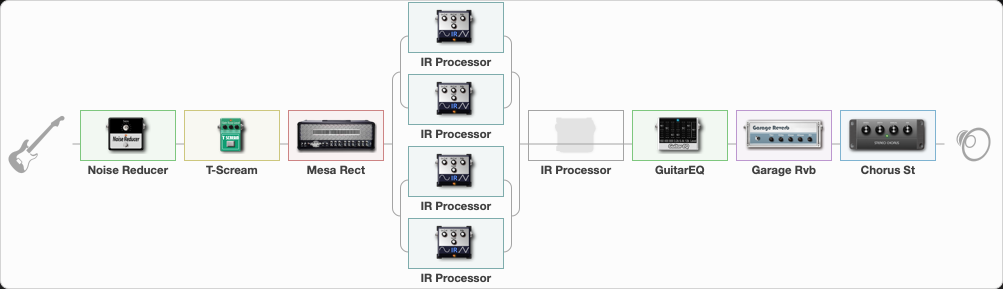# Megadeth - Rust In Peace

Discussion in 'ToneLib-GFX presets' started by grigoriy0192873, Mar 20, 2023.

1. ### grigoriy0192873Active Member

Preset name: Rust in Peace

Effects chain:Effect: "Noise Reducer" (Dynamics / Filter), active - "yes"
{
"Sens" = 60
"Mode" = Hard
}

Effect: "T-Scream" (Overdrive / Distortion), active - "yes"
{
"Drive" = 0
"Tone" = 100
"Level" = 23
}

Effect: "Mesa Rect" (Amp simulators), active - "yes"
{
"Gain" = 66
"Bass" = 57
"Middle" = 88
"Treble" = 84
"Presence" = 81
"Master" = 30
"Level (dB)" = 10
}

Effect: "Splitter" (Dynamics / Filter)
{
"A-Bypass" = Off
"A-Pan" = -25
"A-Level" = 55
"B-Bypass" = Off
"B-Pan" = 25
"B-Level" = 55
"Width" = 0

'A' branch:
{

Effect: "Splitter" (Dynamics / Filter)
{
"A-Bypass" = Off
"A-Pan" = -25
"A-Level" = 55
"B-Bypass" = Off
"B-Pan" = 25
"B-Level" = 55
"Width" = 0

'A' branch:
{

Effect: "IR Processor" (Cabinets), active - "yes"
{
"IR" = ASEM RECTO V30 L2
"Low Cut (Hz)" = 70
"Hi Cut (kHz)" = 10.0
"Mix" = 100
"Level (dB)" = 4
}
}
'B' branch:
{

Effect: "IR Processor" (Cabinets), active - "yes"
{
"Low Cut (Hz)" = 0
"Hi Cut (kHz)" = 20.0
"Mix" = 100
"Level (dB)" = 0
}
}
}
}
'B' branch:
{

Effect: "Splitter" (Dynamics / Filter)
{
"A-Bypass" = Off
"A-Pan" = -25
"A-Level" = 55
"B-Bypass" = Off
"B-Pan" = 25
"B-Level" = 55
"Width" = 0

'A' branch:
{

Effect: "IR Processor" (Cabinets), active - "yes"
{
"IR" = ASEM RECTO V30 L2
"Low Cut (Hz)" = 70
"Hi Cut (kHz)" = 10.0
"Mix" = 100
"Level (dB)" = 4
}
}
'B' branch:
{

Effect: "IR Processor" (Cabinets), active - "yes"
{
"IR" = Rust in Peace - IR
"Low Cut (Hz)" = 0
"Hi Cut (kHz)" = 20.0
"Mix" = 100
"Level (dB)" = 0
}
}
}
}
}

Effect: "IR Processor" (Cabinets), active - "no"
{
"IR" = ASEM RECTO V30 L2
"Low Cut (Hz)" = 70
"Hi Cut (kHz)" = 10.0
"Mix" = 100
"Level (dB)" = 4
}

Effect: "GuitarEQ" (Dynamics / Filter), active - "yes"
{
"160 Hz" = 1
"400 Hz" = -1
"800 Hz" = 0
"1.6 kHz" = 0
"3.2 kHz" = 0
"6.4 kHz" = 2
"12 kHz" = -3
"Level (dB)" = 0
}

Effect: "Garage Rvb" (Reverberation), active - "yes"
{
"Time" = 5.2
"PreDelay" = 46
"LoDamp" = 0
"HiDamp" = 30
"Mix" = 10
}

Effect: "Chorus St" (Modulation / Sfx), active - "yes"
{
"Speed" = 2.0
"Depth" = 10
"Center" = 4.0
"Mix" = 40
}

Note: You will need to download and install the ToneLib-GFX software to use the preset.